# C.4 The covariance matrix

The terms of the covariance matrix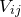are defined by: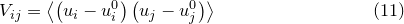(C.8)

Its leading diagonal terms may be recognised as equalling the variances of each of our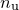variables; its cross terms measure the correlation between the variables. If a component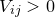, it implies that higher estimates of the coefficient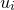make higher estimates ofmore favourable also; if, the converse is true.

It is a standard statistical result that. In the remainder of this section we prove this; readers who are willing to accept this may skip onto Section C.5.

Using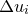to denote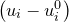, we may proceed by rewriting Equation () as: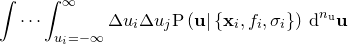(C.9)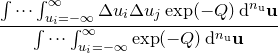The normalisation factor in the denominator of this expression, which we denote as, the partition function, may be evaluated by-dimensional Gaussian integration, and is a standard result: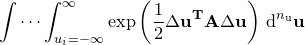(C.10)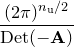Differentiating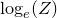with respect of any given component of the Hessian matrixyields: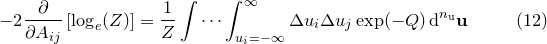(C.11)

which we may identify as equalling: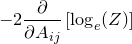(C.12)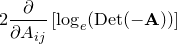This expression may be simplified by recalling that the determinant of a matrix is equal to the scalar product of any of its rows with its cofactors, yielding the result: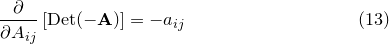(C.13)

whereis the cofactor of. Substituting this into Equation () yields:(C.14)

Recalling that the adjoint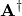of the Hessian matrix is the matrix of cofactors of its transpose, and that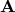is symmetric, we may write: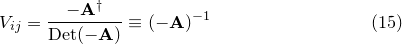(C.15)

which proves the result stated earlier.